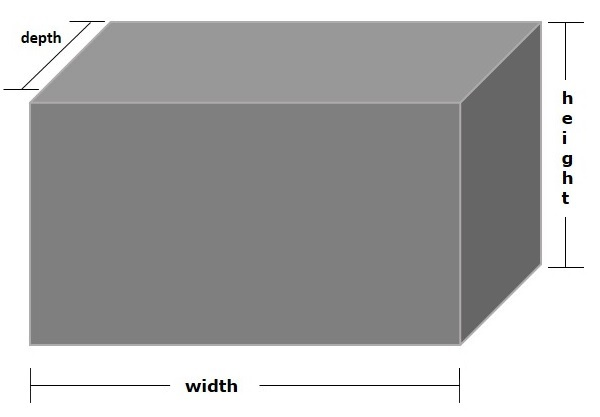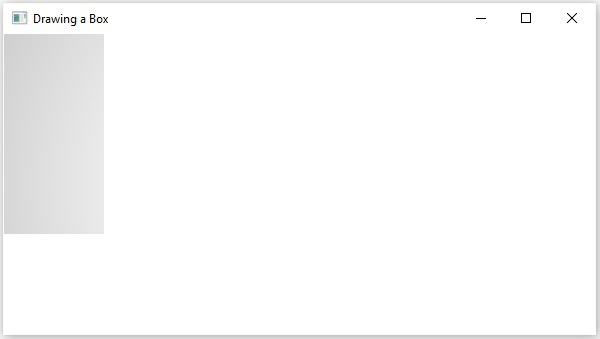# JavaFX - 3D Shape Box

A cuboid is a three dimensional or solid shape. Cuboids are made from 6 rectangles, which are placed at right angles. A cuboid that uses square faces is a cube, if the faces are rectangles, other than cubes, it looks like a shoe box.

A cuboid is a three-dimensional shape with a length (depth), width, and a height as shown in the following diagram −In JavaFX, a 3-dimensional box is represented by a class named Box. This class belongs to the package javafx.scene.shape.

By instantiating this class, you can create a Box node in JavaFX.

This class has 3 properties of the double datatype, which are −

• width − The width of the box.

• height − The height of the box.

• depth − The depth of the box.

To draw a cubic curve, you need to pass values to these properties by passing them to the constructor of this class. This has to be done in the same order at the time of instantiation as shown below −

```Box box = new Box(width, height, depth);
```

Or, by using their respective setter methods as follows −

```setWidth(value);
setHeight(value);
setDepth(value);
```

## Steps to Draw 3D Box

To Draw a 3D box in JavaFX, follow the steps given below.

### Step 1: Creating a Class

Create a Java class and inherit the Application class of the package javafx.application and implement the start() method of this class as follows −

```public class ClassName extends Application {
@Override
public void start(Stage primaryStage) throws Exception {
}
}
```

### Step 2: Creating a Box

You can create a Box in JavaFX by instantiating the class named BOX, which belongs to a package javafx.scene.shape. You can instantiate this class as follows.

```//Creating an object of the class Box
Box box = new Box();
```

### Step 3: Setting Properties to the Box

Set the properties of the 3D box, Width, Height and Depth, using their respective setter methods as shown in the following code block.

```//Setting the properties of the Box
box.setWidth(200.0);
box.setHeight(400.0);
box.setDepth(200.0);
```

### Step 4: Creating a Group Object

In the start() method, create a group object by instantiating the class named Group, which belongs to the package javafx.scene.

Pass the Box (node) object, created in the previous step, as a parameter to the constructor of the Group class. This should be done in order to add it to the group as follows −

```Group root = new Group(box);
```

### Step 5: Creating a Scene Object

Create a Scene by instantiating the class named Scene, which belongs to the package javafx.scene. To this class, pass the Group object (root), created in the previous step.

In addition to the root object, you can also pass two double parameters representing height and width of the screen along with the object of the Group class as follows −

```Scene scene = new Scene(group ,600, 300);
```

### Step 6: Setting the Title of the Stage

You can set the title to the stage using the setTitle() method of the Stage class. The primaryStage is a Stage object, which is passed to the start method of the scene class as a parameter.

Using the primaryStage object, set the title of the scene as Sample Application as follows.

```primaryStage.setTitle("Sample Application");
```

### Step 7: Adding Scene to the Stage

You can add a Scene object to the stage using the method setScene() of the class named Stage. Add the Scene object prepared in the previous steps using the following method −

```primaryStage.setScene(scene);
```

### Step 8: Displaying the Contents of the Stage

Display the contents of the scene using the method named show() of the Stage class as follows.

```primaryStage.show();
```

### Step 9: Launching the Application

Launch the JavaFX application by calling the static method launch() of the Application class from the main method as follows −

```public static void main(String args[]){
launch(args);
}
```

### Example

Following is a program which generates a 3D box using JavaFX. Save this code in a file with the name BoxExample.java.

```import javafx.application.Application;
import javafx.scene.Group;
import javafx.scene.Scene;
import javafx.scene.shape.Box;
import javafx.stage.Stage;

public class BoxExample extends Application {
@Override
public void start(Stage stage) {
//Drawing a Box
Box box = new Box();

//Setting the properties of the Box
box.setWidth(200.0);
box.setHeight(400.0);
box.setDepth(200.0);

//Creating a Group object
Group root = new Group(box);

//Creating a scene object
Scene scene = new Scene(root, 600, 300);

//Setting title to the Stage
stage.setTitle("Drawing a Box");

stage.setScene(scene);

//Displaying the contents of the stage
stage.show();
}
public static void main(String args[]){
launch(args);
}
}
```

Compile and execute the saved java file from the command prompt using the following commands.

```javac BoxExample.java
java BoxExample
```

On executing, the above program generates a JavaFX window displaying a 3D Box as shown below −javafx_3d_shapes.htm